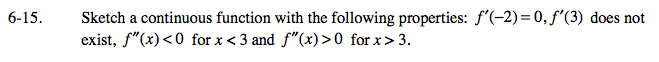### Home > CALC > Chapter 6 > Lesson 6.1.1 > Problem6-15

6-15.f '(−2) = 0

Translation: the slope of f(x) is zero at x = −2. Note: x = −2 is a CANDIDATE for local max or min. (It might also be a point of inflection.)

f '(3) = DNE

Reasons why a derivative might not exist at a point:
cusp
endpoint
jump
hole
vertical tangent

f ''(x) < 0 for x < 3 and f ''(x) > 0 for x > 3

Translation: There is a change in concavity at x = 3.